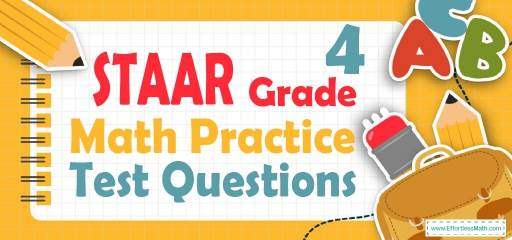# 4th Grade STAAR Math Practice Test QuestionsPreparing your student for the 4th Grade STAAR Math test? Help your students build STAAR Math test skills by following common 4th Grade STAAR Math questions. Practicing common math questions is the best way to help your students improve their Math skills and prepare for the test. Here, we provide a step-by-step guide to solve 10 common STAAR Math practice problems covering the most important math concepts on the 4th Grade STAAR Math test.

## 10 Sample 4th Grade STAAR Math Practice Questions

1- What is the value of A in the equation $$64 ÷ A = 8$$

A. 2

B. 4

C. 6

D. 8

2- Jason’s favorite sports team has won 0.62 of its games this season. How can Jason express this decimal as a fraction?

A. $$\frac{6}{2}$$

B. $$\frac{62}{10}$$

C. $$\frac{62}{100}$$

D. $$\frac{6.2}{10}$$

3- Use the models below to answer the question.

Which statement about the models is true?

A. Each shows the same fraction because they are the same size.

B. Each shows a different fraction because they are different shapes.

C. Each shows the same fraction because they both have 3 sections shaded.

D. Each shows a different fraction because they both have 3 shaded sections but a different number of total sections.

4- Sophia flew 2,448 miles from Los Angeles to New York City. What is the number of miles Sophia flew rounded to the nearest thousand?

A. 2000

B. 2400

C. 2500

D. 3000

5- Write $$\frac{124}{1000}$$ as a decimal number.

A. 1.24

B. 0.124

C. 12.4

D. 0.0124

6- Erik made 12 cups of juice. He drinks 3 cups of juice each day. How many days will Erik take to drink all of the juice he made?

A. 2 days

B. 4 days

C. 8 days

D. 9 days

7- Jason has prepared $$\frac{4}{10}$$ of his assignment. Which decimal represents the part of the assignment Jason has prepared?

A. 4.10

B. 4.01

C. 0.4

D. 0.04

8- Emma described a number using these clues.
• 3 digits of the number are 4, 7, and 9
• The value of the digit 4 is $$(4 × 10)$$
• The value of the digit 7 is $$(7 × 1000)$$
• The value of the digit 9 is $$(9 × 10000)$$
Which number could fit Emma’s description?

A. 9,724.04

B. 90,734.40

C. 97,040.04

D. 98,740.70

9- There are 18 boxes and each box contains 26 pencils. How many pencils are in the boxed-in total?

A. 108

B. 208

C. 468

D. Not here

10- Emily and Ava were working on a group project last week. They completed $$\frac{7}{10}$$ of their project on Tuesday and the rest on Wednesday.
Ava completed $$\frac{3}{10}$$ of their project on Tuesday. What fraction of the group project did Emily complete on Tuesday?

A. $$\frac{2}{10}$$

B. $$\frac{3}{10}$$

C. $$\frac{4}{10}$$

D. $$\frac{5}{10}$$

## Best 4th Grade STAAR Math Prep Resource for 2022

1- D
$$A = 64 ÷ 8$$
$$A=8$$

2- C
0.62 is equal to $$\frac{62}{100}$$

3- D
the model for the first fraction is divided into 6 equal parts. We shade 3_6 to show the same amount as 1_2. The model for the second fraction is divided into 8 equal parts. We shade 3_ 8 that it shows these two models are different fractions.

4- A
When rounding to the nearest thousand, you will need to look at the last three digits. If the last three digits is 449 or less round to the next number that is smaller than the number given and end with three zeros.
On the other hand, If the last three digits is 500 or more, round to the next number bigger than the given number and end with three zeros.

5- B
$$\frac{124}{1000}$$ is equal to $$0.124$$.

6- B
$$12 ÷ 3 = 4$$

7- C
$$\frac{4}{10}=0.4$$

8- C

9- C
$$18 × 26 = 468$$

10- C
$$\frac{7}{10}-\frac{3}{10}=\frac{4}{10}$$

## The Best Books to Ace 4th Grade STAAR Math Test

### What people say about "4th Grade STAAR Math Practice Test Questions - Effortless Math: We Help Students Learn to LOVE Mathematics"?

No one replied yet.

X
30% OFF

Limited time only!

Save Over 30%

SAVE $5 It was$16.99 now it is \$11.99